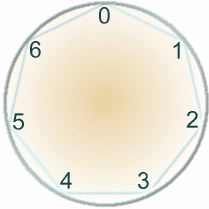#### You may also like### Differences

Can you guarantee that, for any three numbers you choose, the product of their differences will always be an even number?### Two Much

Explain why the arithmetic sequence 1, 14, 27, 40, ... contains many terms of the form 222...2 where only the digit 2 appears.### Where Can We Visit?

Charlie and Abi put a counter on 42. They wondered if they could visit all the other numbers on their 1-100 board, moving the counter using just these two operations: x2 and -5. What do you think?

# Days and Dates

##### Age 11 to 14Challenge LevelIf today is Monday we know that in 702 days' time (that is in 100 weeks and 2 days' time) it will be Wednesday. This is an example of "clock" or "modular" arithmetic.

What day will it be in 15 days? 26 days? 234 days?

In 2, 9, 16 and 23 days from now, it will be a Wednesday.

What other numbers of days from now will be Wednesdays?
Can you generalise what you have noticed?

Choose a pair of numbers and find the remainders when you divide by 7.
Then find the remainder when you divide the total by 7. For example:

 $15 \div 7 = 2$ remainder $1$ $15 + 26 = 41$ $26 \div 7 = 3$ remainder $5$ $41 \div 7 = 5$ remainder $6$

Choose some more pairs of numbers.
Is there a relationship between the remainders when you divide each by 7, and the remainder when you divide their total by 7?

Now find the remainder when you divide the product of 15 and 26 by 7. What happens?

Choose some more pairs of numbers.
Is there a relationship between the remainders when you divide each by 7, and the remainder when you divide their product by 7?

What about when you divide by numbers other than 7?
Can you explain what you've noticed?

How could you use these ideas to work out on which day of the week your birthday will be next year?